# Lindelöf hypothesis

(diff) ← Older revision | Latest revision (diff) | Newer revision → (diff)

Lindelöf conjecture, on the behaviour of the Riemann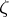-function

For any,It was stated by E. Lindelöf . The Lindelöf conjecture is equivalent to the assertion that for a fixedthe number of zeros of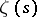that lie in the domain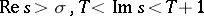is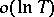. The Lindelöf conjecture is therefore a consequence of the Riemann conjecture on the zeros of(cf. Riemann hypotheses). It is known (1982) that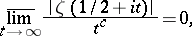whereis a constant such that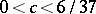.

There is a generalization of the Lindelöf conjecture to Dirichlet-functions: For any,whereis the modulus of the character.

How to Cite This Entry:
Lindelöf hypothesis. Encyclopedia of Mathematics. URL: http://encyclopediaofmath.org/index.php?title=Lindel%C3%B6f_hypothesis&oldid=18908
This article was adapted from an original article by S.M. Voronin (originator), which appeared in Encyclopedia of Mathematics - ISBN 1402006098. See original article